# A Magical Theorem: Antiderivatives and Areas, Part I

Sometimes in physics, we need to add up lots of little things.  In fact, an infinite number of infinitesimally small things.  When faced with this challenge, we use a method that takes advantage of a theorem so helpful and so concise that it feels like magic.

But the way we do this is really quite subtle.  You could master the method in math class and then still have trouble making the jump to using the method in physics class.  So in these next blog posts, I want to sort this out, even if just to help me organize my teaching.

Overview

Part I introduces the idea of an “anti-derivative” and it’s notation.  It also introduces a similar-and-yet-oh-so-different notation for area.  It turns out that when we talk about “integrating”, there are two different but related ideas that we might be referring to.  And we casually jump back and forth between them in a way that could easily blur the distinction.  So in this post, I try to at least make the distinction clear again.

Part II explains the connection between finding areas and finding anti-derivatives.  Essentially, this post introduces the Fundamental Theorem of Calculus (or Magic Theorem)  which gives us an easy way to find areas.  Part II closes by raising the question, “Why do we care so much about finding area in physics?”

Part III steps back and discusses Riemann Sums. This is the method we would have to use to find area if we did not have the Magic Theorem.  We won’t actually use this method, but we need to understand what the method does if we are going to understand “integrating” in physics.

Part IV revisits the question of finding displacement when velocity varies as a function of time.  So we will see one reason to find area (or integrate) in physics.

Part V (the last, I hope) will demonstrate how we use these area-finding methods whenever we need to add up a bunch of tiny little pieces.

OK, here we go…

Part I: “Integrals” — One word, Two meanings

If you have learned a little bit of calculus, even just by working your way through these Summer Reading posts, then at this point, you know how to find “derivatives” for a nice collection of reasonable functions.  Nothing too crazy or exotic — just the kinds of functions that you will meet early in AP Physics C.  And you also know why we find derivatives:  derivatives are slope-finding formulas.

For example:

Velocity is the rate of change of position with respect to time.

Acceleration is the rate of change of velocity with respect to time.

Force is the rate of change of momentum with respect to time.

Current is the rate of change of electric charge with respect to time.

For example, suppose you are given that an object moves so that it’s velocity as a function of time is:

v(t) = t2

You can easily find the acceleration as function of time by taking the derivative:

a(t) = v’(t) = 2t

So there are good, useful reasons for learning how to find derivatives. (In fact, let me remind you that to “optimize” a function, you usually begin by finding the derivative and then setting it equal to zero.)

CAN WE PLAY THE GAME IN REVERSE?

If I give you a function, can you find an “antiderivative”?

For example, let’s say:  f(x)=x2

Can we find another function that has f(x)=x2 as its derivative? If we know the basics, finding this particular antiderivative is not that hard:

F(x)=(1/3)x3

But you don’t have to take my word — go ahead an take the derivative!  You will see for yourself that the derivative of F(x)=(1/3)x3 is indeed f(x)=x2.

(But also, in this case you can see where we got this: taking a derivative lowers the power so we better raise the power by 1.  Oh, and the power will come out in front as a multiplier, so we stick in a factor of 1/3 to cancel that…)

So  F(x)=(1/3)x3 is an antiderivative of  f(x)=x2.

But it is not the only one!  Try taking the derivative of any of these:

F(x)=(1/3)x3 + 5

F(x)=(1/3)x3 – 99

F(x)=(1/3)x3 + C where C is a constant

So if you can find one antiderivative, you can find a whole family of them.  Still, most times, you only need to find one.

[Caution: your math teacher will likely take off a point or two if you leave out the “…+ c” when finding an antiderivative. They are kind of obsessive about this.  ]

IS IT ALWAYS THIS EASY?

Well, no.  Some antiderivatives are easy to find.  But others…not so much.  You will spend a substantial amount of time in math class building a collection methods for finding antiderivatives for an increasing variety of functions.  Some of these methods are quite subtle.  You can easily start to think that this quest for antiderivatives is what it means to do calculus.  But it is really just a means to a more useful end, not the main event.  And in fact, there are even functions that don’t have antiderivatives whose formulas we can find!

For now, I want to make two points:

1. In our AP Physics class, the antiderivatives we need will mostly be pretty easy to find.  You will be able to use “guess and check” to find them.  And if they ARE  hard to find, you can have a little help: your TI89s or N-Spires know how to do this.  So does Wolfram Alpha.

2. Nobody would be willing to invest so much effort in finding antiderivatives unless there was something really useful about them! We will get to that soon. But first:

A NEW NOTATION

If we are going to be using antiderivatives, we should have symbols for writing them.  To indicate that F(x) is the antiderivative of f(x), we write: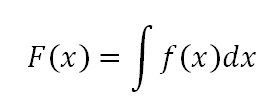This is just another way of saying that F'(x) =  f(x).  And, in addition to calling F(x)  an antiderivative, we also sometimes call it the “indefinite integral of f(x)”.

BUT WAIT…

On the other hand, there is another symbol that looks almost the same but means something completely different:This symbol is called the “definite integral” (as opposed to “indefinite”).  It is not a function at all! Just adding values at the top and bottom of that strange elongated s-shape gives the symbol a whole new meaning:

The definite integral is the area of the region bounded by the function, the x-axis and the two “limits of integration”: the vertical lines defined by x=a and x=b.

For example, here is a definite integral:And here is the area that definite integral is referring to:So now we have two very different ideas that have been given very similar symbols and names. It would be easy to get these two things confused…

There is the INDEFINITE INTEGRAL:This is a FUNCTION which has f(x) as its derivative.

And then there is the DEFINITE INTEGRAL:This is an AREA — a numeric value.

And now the big question:

Why do mathematicians use these similar names and symbols for these two very different ideas?  Are they secretly related in some FUNDAMENTAL way?

That is the topic of the next post, Part II of this discussion.

# “A positive times a negative” and other mysteries of arithmetic

“Is that the kind of thing you just have to memorize?”

That’s a question that should make a math teacher nervous.  There are certainly procedures and formulas that are occasionally useful to memorize.  But not “just” memorize!  If we don’t aim for a deeper understanding, then we have lost sight of why we are doing any of this.  In fact, if we never even try to have our students understand some particular piece of mathematics, then that piece is a good candidate to drop from the curriculum. (I’m looking at you, Descartes Rule of Signs).

On the other hand, we do want our students to be able to do a lot of things!  And the reasons behind some of them are sometimes more subtle or more advanced than what students need to hear at the moment they are first learning them.  So it can be a bit of a balancing act — finding the right degree of explanation and rigor at every level.

One somewhat disheartening thing I see at the high school level is that my students don’t seem to expect to understand the rules.  They have been graded on their ability to replicate a startling number of procedures.  That these things can be understood seems almost beside the point to them.  I wish we taught a whole lot more about a whole lot less…

So what has me started on this rant on a lovely snow day in the new year?  Well, while not grading a pile of papers, I came across a twitter discussion of this question:

Why is a negative times a negative a positive?

As you ponder this issue, you see that it is related to:

Why is a positive times a negative a negative?

I would like to offer an answer that requires no algebra — so it can be used with the students who are first learning these rules.

GUIDING IDEA

Often in math, we start with an idea that has one interpretation and then we extend it into new areas that cause us to expand or even give up the original interpretation. But we try to extend the idea in ways that continue the rules and patterns that we had before.

So for example, when you first learned to multiply, say 5 × 4 = ?

You can think of that as 5 sets of 4.  (Or 4 sets of 5!)

You can make rows of dots:

•    •    •    •

•    •    •    •

•    •    •    •

•    •    •    •

•    •    •    •

But now someone asks you to multiply a positive times a negative: 5 × -4 = ?

I don’t know how to make 5 rows with -4 dots in a row.  Or -4 rows with 5 dots in each.  So to answer this question, I am going to have to extend what it means to multiply.  But I want to keep patterns intact.  What kind of patterns? Well, here’s one:

5 × 4 = 20

5 × 3 = 15

5 × 2 = 10

5 × 1 = 5

5 × 0 = 0

Hmm.  When you are multiplying 5 by successively smaller whole numbers, your answer decreases by 5 each time.  What if we want that pattern to continue?

5 × -1 = -5

5 × -2 = -10

5 × -3 = -15

5 × -4 = -20  Aha!  That was the question we started with.  We just found a way to multiply a positive by a negative.

[If all you care about is getting the answer, then you memorize the procedure: to multiply a positive by a negative, just multiply the “regular” way and then make your answer negative.  But without some understanding, it feels so hollow.]

But wait!  There’s more…

Now someone asks you to multiply, say:  -4 × -5 = ?

I still don’t know how to draw -5 rows each with -4 dots.  But I can look for patterns!

We have learned how to multiply positives and negatives. So for example…

4 × -5 = -20

3 × -5 = -15

2 × -5 = -10

1 × -5 = -5

0 × -5 = 0

Any pattern?  Hmmm.  When you multiply -5 by successively decreasing whole numbers, the products INCREASE by 5 each time…and if we continue the pattern:

-1 × -5 = +5

-2 × -5 = +10

-3 × -5 = +15

-4 × -5 = +20  Aha (again)!  We have our answer — not because we “just” memorized that a negative times a negative is a positive, but because that is the way it has to be for our pattern to be extended.

The idea of developing new rules in a way that maintains old patterns will certainly be useful again. In fact, I just remembered another example of this.  I once gave a guest lesson to a 5th grade class that wanted to know why a number raised to the power of zero is 1.  I came up with a kind of lengthy explanation involving the laws of exponents…I don’t know how many hearts and minds I won that day.  Then I went home and thought about it a little more.  I came back the following day with a much shorter lesson:

2^5 = 32

2^4 = 16

2^3 = 8

2^2 = 4

2^1 = 2

What happens to the answer each time you lower the exponent by 1?  Keep that pattern going…

# That Trigonometry Diagram Again

Since I started this blog, one recurring goal has been to explore ways to visualize mathematical ideas.  In that spirit,  I spent a few posts talking about this diagram here: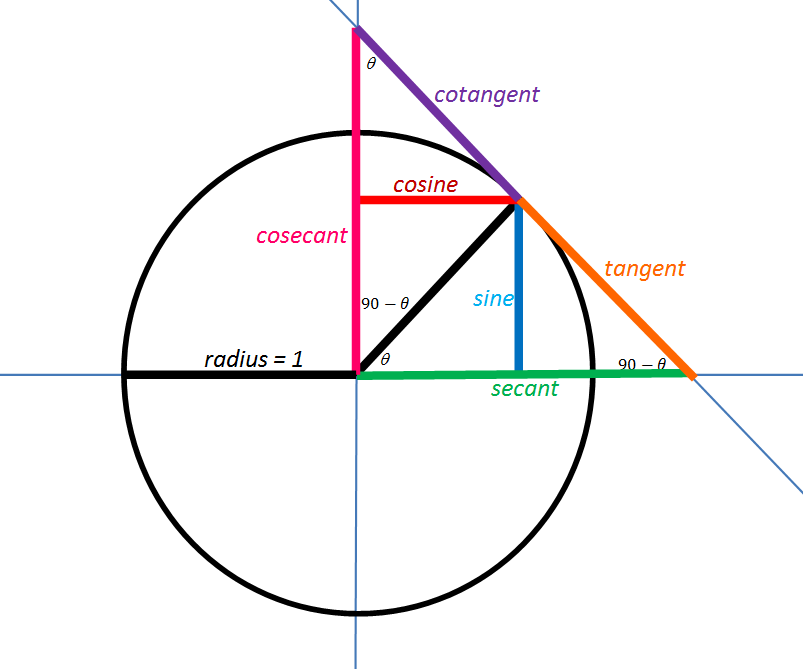These three posts discuss the development of this diagram:

Also Better With Pictures — The Trigonometry Ratios

Picturing the Trigonometric Ratios: Step-by-step

Trig Pictures, Part III

Whenever I show this diagram to students, they seem actually excited to discover the meaning and reasons behind so much trigonometry that they had previously just memorized.  It makes me believe that it would be helpful if this diagram was displayed in every room where trigonometry is introduced.  And now it can be!  I tidied up the graphics and made it into a poster.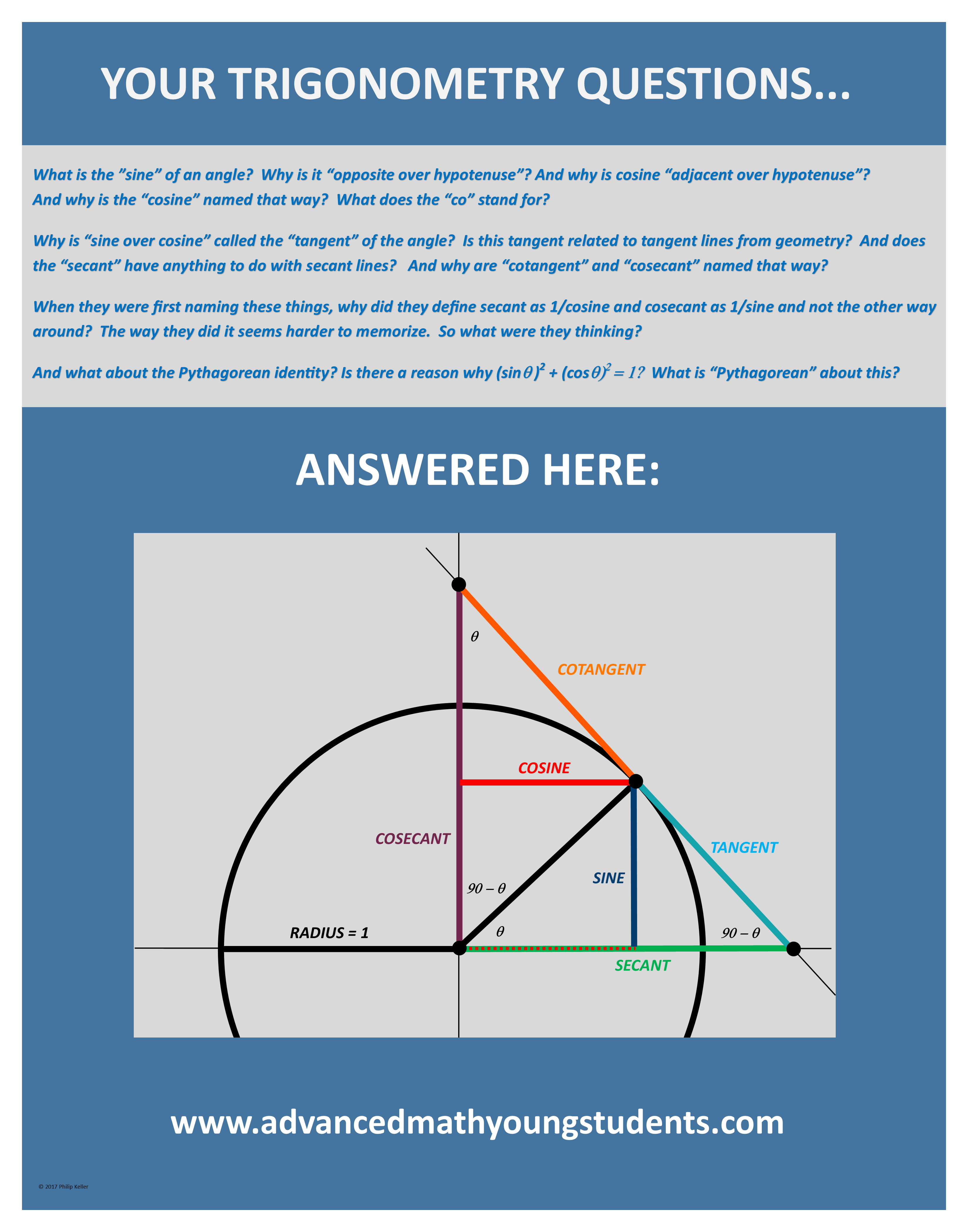For your notebook (8.5″ x 11″)  Trig Diagram Notebook-sized

For your wall (22″ x 28″ ) Trig Diagram Poster-Sized

COMING SOON: T-SHIRTS!!  (Maybe)

UPDATE 11/1/2017 My obsession continues…now you can see an animated version.  I made this in Desmos.  You can see it here:

https://www.desmos.com/calculator/mvj7yljovi

Now, how do I get THAT on a t-shirt?

# ALTERNATIVE SOLUTIONS: TEST #8

For more than half of the problems on this test, there is another way to solve the problem, a way that is sneakier, more creative, a little subversive.  It’s good to have more than one way to attack a problem, so read on and learn to play.

DITW = Do it their way

Trial and Error = pick an answer, play with it, see if it fits what they said in the problem.

Back Door = make up numbers for the variables in the problem, work out an answer that is based on the numbers you made up, then put those made up numbers back into the answer choices, ruling out any that don’t produce a matching answer.

More detailed explanations available in my book

PRACTICE TEST #8, SECTION 3: NO CALCULATOR

1. As algebra goes, this is not that bad. But you can also just try the answers. Lazy kids try b first  but no luck. Then they try d and their laziness is rewarded.
1. Well, you should REALLY know how to find a linear equation from a graph. So I guess I am saying DITW. But you can also pick a point on the graph,  say (1,2)  and then see which equation works. If you are unlucky and two answers work (as would happen if you chose m=2, d=4) then pick another point and try again.
1. If you can do the algebra to solve for P, go ahead. But this is also a classic back door problem.  Make up #s that fit, get your answer and then plug your #s into the answer choices.
1. DITW
1. And another back door!  Draw a rectangle, pick a value for w, make the length 6 more than that, find the perimeter and then stick your w number into each answer.
1. DITW
1. Why do algebra?  Try the numbers! 5 works,  -1 does not.  No need to check 0 (but it doesn’t work either).
1. And yet another back door! Try x = 4, see what you get.  Then plug x=4 into each answer.
1. You do have to know that this is a circle centered at (6,-5).  Draw the picture, fill in the center and the endpoint that they gave you: P(10,-5).  Then look at the answers and plot them on your graph.  There will only be one that could be anywhere close to the other end of that diameter.
1. The SAT LOVES this kind of problem.  The official solution shows the algebra method.  They also discuss a slick think-around.  But they forget to mention that you can also use trial and error. For example, say there were 20 2-person tents (B). That takes care of 40 people.  The other 40 of the 60 tents would be 4-person and would hold 160.  That adds up to 200 people, but we need 202.  I bet choice C will work. Try it!
1. If I plug in x=-2, the graph tells me to expect an output around almost -20…try the answer choices.
1. Make up an a and a b that fit: for example, a=1, b=4 — in other words, this is yet another back door problem!
1. While I still think you should know how to write a linear function, note that the right answer has to give an output of 1.9 when the input is 13. So check the answers to see which one does what it’s supposed to.
1. DITW
1. DITW
1. Before doing algebra, a lazy kid checks 1,2 3 and maybe even -1, -2 and -3. Oh, look: 3 works
1. DITW
1. DITW (but they say it funny: just add the equations, lined up as they are.  You get 4y=6, so y=1.5
1. They LOVE this question too: y=ax+b and a is the rate of change, b is the initial value.  If you know that, this is kind of easy to be problem 19.
1. DITW

PRACTICE TEST #8, SECTION 4: WITH CALCULATOR

1. Back door  say c=5. That’s \$10. Now put c=5 into the answers…
1. DITW
1. This is a classic ratio problem: set up thing1/ thing 2 = other thing 1/other thing 2.

4. While you COULD plug x=19 into the equation they give you, you could also estimate directly from the graph.  You have to extend the line a little bit but the answer is clearly a little more than 50 and clearly less than 55.

1. DITW
1. Since you don’t actually have to solve the system, it’s better to DITW.
1. Y=mx+b and the slope is 3.
1. If you see that you can multiply both sides by x+1 to get (x+1)^2, then you take the square root of both sides. That’s their way.  You could also do trial and error.  Or, since this is the calculator section, if you have a ti89 or an nspire, you can let the calculator do the algebra for you.
1. You have a calculator!  They give you a formula.  And they give you answers! Try them one at a time.  When you get 473, stop.
1. An odd and interesting question.  But DITW.
1. Division, or just trial and error…
1. DITW
1. This will be an easy back door play as long as you know what a negative exponent means and what ½ as an exponent means. When it says a ^(-1/2) that means “one over the square root of a”.  So if you make a=25, x will be 1/5.  Now go to the answer choices, plug in 1/5 and see which comes out as 25.
1. It will be undefined when the denominator = 0. But you can skip the algebra and just try the answer choices.
1. DITW
1. DITW  but again I have to complain about the wording.  They say “what proportion” when they should really say “what fraction”.  I know I am nitpicking, but still…
1. If you understand their way that’s fine. But many people find it easier to understand if they list out all the data:

18,18,18,18,18,18, 19, 19, 19, 19, 19, 20,20,20,20,20, 21,21,22,23,30

Notice how much easier it is now to find mean, median and mode.

1. DITW
1. DITW
1. DITW
1. Yet another question testing your ability to interpret y=mx+b. The m is the slope which is also the rate of change.
1. DITW
1. I am sure that many students who think they are too advanced to bother making up numbers will actually get this wrong (by putting b). Say t=3. Calculate 1800(1.02)^3.

In 3 years, there are  q=12 quarters.  Put q=12 into each answer choice.  You will see that it is a and not b that’s the answer you were looking for.

24. Well, DITW…but also: you get this wrong by assuming things that the problem never said.  In the math section, as in the reading section, that will burn you every time.

1. Yes, you could find the slope and intercept and then write the equation…but here’s something helpful to notice: when you plug t = 10 into the right answer, you better get 846 back as your answer.  Start calculating!
1. DITW
1. DITW and note that this is a righteously challenging question, as it should be for its location in the section.
1. You do not need to know how to calculate standard deviations. You just need an intuitive feeling that if the data is clustered around the mean, the std. deviation is low and if it is spread out, the std deviation is higher. Then, you can just eyeball this one.
1. First of all, eliminate c and d — they are exponential but the problem says “constant rate” so you know we need linear graphs. And after 20 minutes, 30% of the paper is gone so 70% remains or 3500 sheets. So of the two answers, pick the one that comes out to 3500 when you plug in m = 20.
1. DITW and it’s a good problem!
1. Remember: early grid-in should be easy.  Don’t be psyched out by the chemistry.  You have 51 thingies.  They each contain two smaller thingies of one kind and one of a second kind.  So overall, you have 102 of the first kind and 51 of the second kind.
1. Stick in x=1. Algebra or trial and error to find what works.
1. If you multiply both sides of an equation by a constant, it’s an equivalent equation with the same solutions and the same graph!  It’s easy to see that the constant they multiplied by this time was 3. So 10 x 3 = 30.
1. DITW
1. Yes, you can set the two equations equal and do the algebra.  Or you can let your ti89 do it.  Or with any graphing calculator, you can graph both equations and then trace along until you find the intersection.  But my laziest students draw the g function’s graph by hand! You know it starts at (0,10) and it has a slope of -1. So go to (0,10). Then,go down 1, over 1, down 1, over 1 (watching the scale of the graph). In two steps, you will land on the first intersection point.
1. This is exactly the kind of question that rewards thorough SAT prep. It looks very hard.  But then you notice that the angles they ask about are complementary. And you know the rule for complementary angles: sine of one = cosine of the other. So they subtract to get zero.  If you take 5 practice tests, you will see this concept tested 3 times.
1. Just read the chart and be careful: for the first sample, you multiply by 10, for the second you multiply by 8 and then you subtract the two results. (Which is, to be fair, DITW)
1. And this is DITW as well.

OK, I count more than 30 out of the 58 that have at least one alternative solution!

# Exponential Decay and Time Constants

The other day, a colleague showed me a diagram about current vs. time in an RC circuit. The diagram helped me realize that there is another way to think about time constants and exponential decay, a way I had completely overlooked.  I had all the right pieces floating around but I had never put them together.

WHAT’S A TIME CONSTANT?

Here are three graphs showing a quantity that is decaying exponentially toward zero: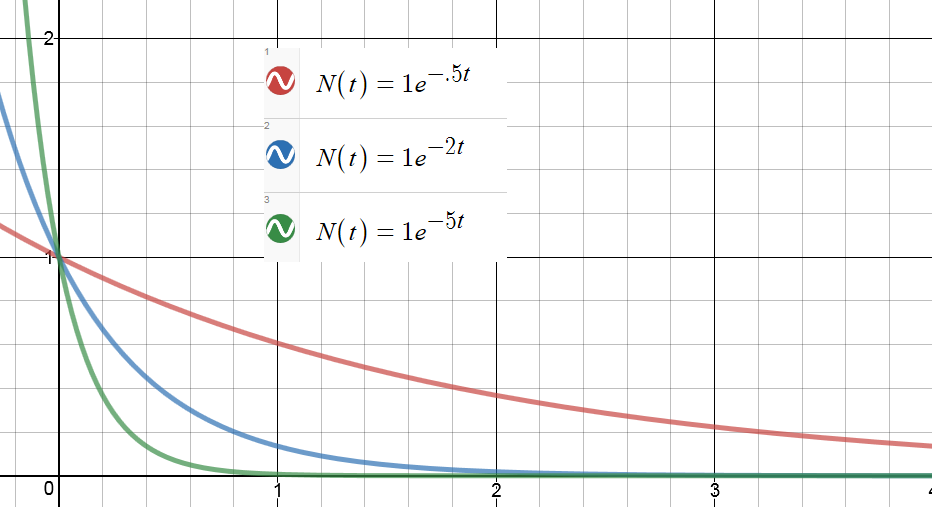Each of these is a graph of a function: N(t) = Noe-kt

The only difference is the value of the constant, k.  Higher values of k lead, in a sense, to faster decay.

To help emphasize this, we can define a constant: τ = 1/k

Then we can  re-write the function this way: N(t) = Noe-t/τ

We call τ the “time constant” for this decay.  It has the units of time.  And it gives us an intuitive feeling for how fast a function is decaying.

For every time constant that passes, our decaying quantity gets reduced by another factor of e.

So after one time constant has passed, the function’s value is No/e.  After two time constants, it’s No/e2.  After three, No/e3 …and so on.

But there is another way to think about the time constant.

The time constant tells us how long it would take to reach the asymptote at the current rate of change.

Maybe this should have been obvious, but I never thought about that way until just a few days ago. Here’s the diagram that got me started:The claim here is made in the context of a charging capacitor, but it is actually true for any function that is approaching an asymptote exponentially. Here is an animation I made in Desmos to illustrate the point:

In the still picture above, you can see the tangent line at t=0.  The dotted green segment on the t-axis represents the time it would take for the tangent line to reach the asymptote.  When you run the video, you will see the function decrease and the tangent line get shallower.  But that green segment stays the same length.

(If you want to play with the Desmos file, you can get it here🙂

WAIT – WHAT’S A TIME CONSTANT NOW?

A quantity that decays exponentially approaches its final value asymptotically. And as it decays, its rate of decay decreases as well.  (That’s the defining characteristic of exponential decay: the rate of decay toward the final value is proportional to the current distance from that value.  In fact, we can write that as a differential equation. I went on and on about that here.)

But now suppose that at some moment, the decay rate were to become constant. Instead of taking forever, the function would now be able to reach its asymptote in a finite amount of time. Not in just any old amount of time, but in fact in an amount that equals the “time constant” of the decay. It doesn’t matter what moment you pick.  From any starting point during exponential decay, if the rate were to stay constant (which it doesn’t, but still…) the time to reach the asymptote would always comes out to τ = 1/k.

Or to say the same thing geometrically: the tangent line from any point on the curve will intercept the asymptote after one time constant has elapsed. That’s what the animation is trying to emphasize.  I chose a k value of .2 so my time constant was 1/.2 = 5 seconds.  If you open the Desmos file, you can change the constants to see what changes and what doesn’t.

SO WHY DID THIS SURPRISE ME?

I wasn’t making the connection between the exponential decay and the differential equation lurking in the background (even though I teach that connection and have blogged about it).

That the function decreases with a rate of change that is proportional to the value of the function is actually a first-order differential equation.  The exponential decay is its solution. (Again, gory detail here.)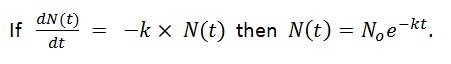And if slope is rise over run, then run is rise over slope!  When the function is starting from a higher value, the tangent has a slope that is PROPORTIONALLY higher!  So that proportionally higher slope will get you back to the asymptote in the same amount of time every time.

To find how much time we are talking about, divide the “rise” (or in this case, a “fall”) by the slope: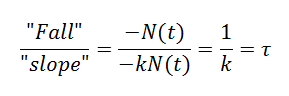I’ll leave a more rigorous, math-y proof as an exercise for those of you in your first calculus class.

# James Tanton is Still Rolling

“If I roll a die five times, how many distinct values should I expect to see?”

I was feeling pretty good about myself having solved the first one. (Here) And my Excel simulation told me I was at least close.  But when I attacked this new one, I hit some trouble.  I know I need the expected values, but to get them, I need the probabilities.  And I was bogging down in the calculations.  I could find the probability of getting one distinct value, but two was harder, three harder still…

Simulate first and calculate later

I already had the spreadsheet with the random integers.  But Excel does not have a “number of discrete values on the list” function.  So even there, I was stuck.

[Side note: back in the day when I did know how to code, I briefly knew an obscure programming language called APL.  I believe that in APL, a problem like this can be solved in a single line of code, dense with obscure symbols.  Document your code, campers, or you will never remember what you did!]

But since JT only rolled the dice 5 times, I did eventually come up with a way to have Excel do this for me. Let’s call it the “Go Fish” procedure, naming it after the simple card game.  It’s not very elegant but it works and, as you will see, it pays extra dividends.

For each possible dice value, 1 through 6, I made a column that answered the question: did this value appear on the list. For example, the formula in my first column answers the Go Fish question: got any ones?

=1×or((a2=1),(b2=1),(c2=1),(d2=1),(e2=1))

This generates a value of 1 if any of the dice came up as a 1 and zero if none of them did.

I made a total of 6 columns like this. Then, the sum of those columns tells me how many distinct values appeared in my original 5 rolls of the dice.

From there, it was just a matter of doing this in every row and taking the average. Again I did 10 years worth of rows.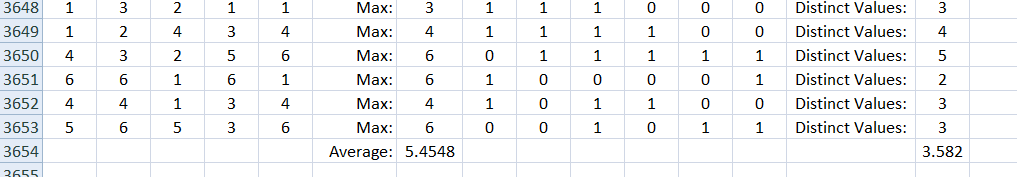You can see in the top row that the dice came up: 1, 3, 2, 1, 1

So the answer was yes if the question was got any 1’s, 2’s or 3’s and no for any 4’s, 5’s or 6’s.  That gave a total of 3 distinct values this time.

OK, so now I have a rough idea of the answer. But aren’t I just stalling?  I should get back to work calculating the probabilities and expected values.

[Really, I am stalling.  I should be grading lab reports.]

Then I realized that the procedure I used to generate the answer in the simulation can be used to calculate the answer directly:

Say you want to know the probability that your list contains a 1. That is more easily calculated as 1 minus the probability that it contains no 1’s.

P(got any ones) = 1 – (5/6)5

But that is also the probability for any of the single Go Fish questions:

P(got any ones) = P(got any twos) = P(got any threes)…and so on.

So the expected value of the total of the “Go Fish” questions = 6 times the value for any one of them!

That means we expect 6×(1 – (5/6)5) = 3.589 different results every time.

That’s not far from what the simulation told me to expect. I ran it 5 more times and got:

3.584, 3.591, 3.586, 3.587, 3.595

So I am feeling like this is a promising answer. I would still like to go back and finish calculating the probabilities the hard way.  But the point to notice here is that taking the time to simulate the problem also provided the key to a solution path.

# James Tanton is on a Roll

A good puzzle is a torment.  And there is something interesting about probability puzzles in particular:  you can’t always tell how tricky they are until you dig into them for a while.  Sometimes a question that seems quite tractable ends up eating up more time and more note paper than you were expecting.

James Tanton has been dropping puzzle after tormenting puzzle on Twitter over these past weeks.  The first one was interesting – and I think I have it solved.  But he was just getting started.

I roll 5 dice every day for a year, recording my high score each day.  At the end of the year, I average those high scores.  What average should I expect?

What we need here is the expected value of the high scores. So first we need the probability of each high score.  Then we can multiply each of those probabilities by its corresponding score.  The sum of those products will be our expected average.

What is the probability of a high score of 1?

Well, you would have to get a 1 every time.  That probability is (1/6)5.  So we can write:

P(high score is 1) = (1/6)5.

What is the probability of a high score of 2?

Now you need a 1 or a 2 every time. That probability is (2/6)5.  But wait!  That includes the cases that turn out to be only 1’s.  But we can subtract the probability we just calculated above to get the probability we want:

P(high score is 2) = (2/6)5  – (1/6)5.

What is the probability of a high score of 3?

We use the same plan: find the probability of getting a high score of as much as 3, subtract the probability of getting a high score of as much as 2  and we will be left with the probability of getting a high score of exactly 3:

P(high score is 3) = (3/6)5  – (2/6)5.

And now we have a pattern to follow:

P(high score is 4) = (4/6)5  – (3/6)5.

P(high score is 5) = (5/6)5  – (4/6)5.

P(high score is 3) = (6/6)5  – (5/6)5.

OK, then we go ahead and multiply each probability by its score and add them up!

When we do, we get an expected value of:

6 – (5/6)5 – (4/6)5 – (3/6)5 – (2/6)5 – (1/6)5 = 5.431

BUT IS THAT RIGHT? Hmm…

I suppose a short computer program could test this. It’s been years since I have written code.  But there’s this program called Excel. And it has a random-integer-generating function. [I used Randbetween(1,6) ]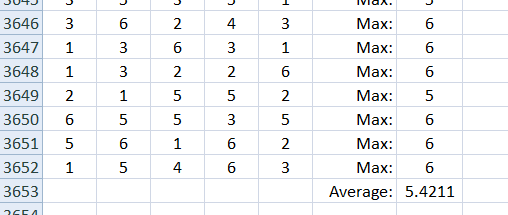As you can see, I actually simulated 10 years of dice rolls. And then I did it again.  And again. And again…The last 5 times I did this, I got 5.4055, 5.4127, 5.4389, 5.4477 and 5.4211.

So I believe I am on the right track. Flush with success, I see on Twitter that Mr. Tanton has posted again:

“If I roll a die five times, how many distinct values should I expect to see?”

Well, how much harder can this be? Ha!  Stay tuned…here it is!

# Alternative Solutions: Pratice Test #7

DITW = Do it their way

Trial and Error = pick an answer, play with it, see if it fits what they said in the problem…

Back Door = make up numbers for the variables in the problem, work out an answer that is based on the numbers you made up, then put those made up numbers back into the answer choices, ruling out any that don’t produce a matching answer.

More detailed explanations available in the book…

PRACTICE TEST #7, SECTION 3 – NO CALCULATOR

1. DITW

2. There way is to distribute and then group terms, which is fine.  But you can also make up a number for x (say x=5), see what you get, and then see which answer choice gives the same value.  In other words, this is actually an entry-level back door problem.

3. If you don’t feel liked doing algebra, just try each answer choice in both equations.

4. DITW

5. DITW

6. You don’t need to do nearly as much algebra as the official answer suggests.  You do need to think about linear functions:  if a linear function takes you from your current value down to zero in 12 years, then in 4 years it will take you 1/3 of the way to zero!  So you will still have 2/3 of the initial value.  [Shouldn’t we be encouraging this kind of thinking?]

7. Another back door:  make up a number for x, get an answer, put that made up number into the answers.

8. Their way is fine, but they say it the slow way.  Also, like many SAT questions, they intentionally give you the info in an inconvenient order.  Start with the fact that 90% of what he earns is \$270.  So without doing algebra, think: what number when reduced by 10% lands you on \$270?  I would not be surprised if \$300 was your first guess.  Then, since he already earned \$80, he needs another \$220.  At \$10/hr, that’s 22 hours.

Also, just for the record, you could also do this one by trying each answer choice one at a time.

9. DITW

10.  This looks like it is going to be much harder than it is!  But it turns out that when they gave you the roots, they also gave you the factors: (x+1), (x+3) and (x-5).  Only one of those is an answer choice.

11. Well, if this were in the calculator section, you could use the back door.  Alas, it isn’t, so you do need to know your exponent laws…DITW

12. Really useful parabola factoid: the vertex lies midway between the zeroes (which they gave you).  Amazingly, that is the way they did it too!  DITW

13. Seriously?  Yet another back door problem: make up a number for x….

14. Still no algebra required.  The lower limit is obviously zero – as it is in each answer choice.  The upper limit can be found by trial and error with the answer choices.  For example, is the width  20?   Well,  2.5 times 20 give a length of 50.  This gives a perimeter of 140 and we have not even added in the height of 60 yet! So 20 is too big…I’d try b next.  Can you see why?

15. OK, start by making up a number for x and getting your value for the expression.  Then, you can either solve for the k value that gives the same value – or use trial and error.  So, if you are keeping score, this problem lets you show off both of the two major algebra-avoidance techniques.  Nice.

16.  When they give you one algebraic expression  and they ask you for another, you should be on the lookout for simple ways to convert one into the other.  In this case, dividing both sides by 2 does the trick.

17. DITW (and who says there isn’t geometry on the new SAT!)

18.  If you only know one thing about radians, know that π radians is 180 degrees.  If you know a second thing about radians, know how to take that fact and scale it up or down.  So if π radians is 180 degrees, 2π radians is 360 degrees, and 4π radians is 720 degrees.

19. All of my students are laughing at this question.  They know that if you just make a really neat drawing, the answer will be staring right at you, no algebra required.

20. DITW

PRACTICE TEST #7, SECTION 4 – WITH CALCULATOR

1. DITW

2. Back door – make up a number…

3. DITW

4. DITW – and this is a common question-type on the new SAT.  Don’t assume more than what the problem actually states.

5. DITW

6. Stick in x = -3. Then, do trial and error with the answer choices.

7. DITW

8. Just read the graph!  At 1.2 on the x axis…

9. As we said earlier, look to transform what they give you into what they ask for.  In this case, you can add 6 to both sides, then divide by 9.  But if you don’t like that, here’s another way: mess around with numbers until you find an a, x and b that make the equation true.  For example, a=2, x=1, b=1…it works!  Then, use those numbers to answer the question!

10. DITW

11. DITW

12. You should learn the fast way to do percent changes: A 6% increase is the same as multiplying by 1.06.  If you knew that, you could just try each answer choice with ease!  Or take the \$53 and divide it by 1.06 to get the initial price.

13. DITW

14. The relevant geometry fact is that the angles of a quadrilateral add up to 360 degrees.  Then, while the algebra is pretty easy this time, you could also do trial and error if you prefer.

15. DITW (Actually, they give two solutions, both fine.)

16.  If you approach this by making up numbers that fit, you will find that you are done in moments.

17. And here it is: another example of y = b + mx.  The b is the flat fee and the m is the rate per mile.  This is a very common question on the new SAT.

18. Once you see what they are asking, this is not that bad.  I’ll translate for you: find the point with the highest y-value.  Then, tell me how far that point is vertically from the line of best fit.

19. They want you to solve for w in terms of A.  But if that gives you trouble, this is also a classic back door problem.  Make up w and A – if you are lazy, try w = 26, A = 1 – then go see which answer works.

20. DITW

21. This is another linear modeling question.  Make sure you know that if y=mx + b (or y=b+mx) the b value is the starting value and the m value is the rate of change.  This time, they are asking for the rate of change, so pick two points and find the slope.

22. This is really just asking if you know how to find a median.  But be careful!  Many students will go right to the middle of the chart and say that the median is 19.5.  That’s a trap: you have to list the data in ascending or descending order and only then can you go to the middle of the list.

23. DITW

24. Well, you can debate whether this is fair or not.  It certainly favors the kids who have had physics.  They would know right away that 72 is the starting height.  You can think of it as the height you get when you plug in t=0.

25. This one is silly.  If you could make up values for x  and k that go together, you could just see which answer works with those numbers. But wait!  You don’t have to make up the numbers – you can pick them right from the chart.  For example, use  x=9 and k=37.7 and see which answer is true.

26.  If you see the algebraic method, that’s fine.  But if not, use the values in the chart to make up numbers for p, f and c so that the total of the calories comes out to 180.  It will take you just a few moments to find a possible set.  Then you can stick those numbers into each answer choice.

27. You should know the quick way to do percent increases and how successive equal percent increases leads to exponential growth.  (See pages 181-183) This is a frequently occurring SAT item. You want to be able to do this about as fast as you can read it.

28. Make up numbers for a point on the line. A line has only one slope.  So far, we know it has risen 6 and run 3.  So keep doing that to find another point.  You could use (6,12).  For that matter, you can even use (3,6). Then, check out the ratio of t to s using your point.

29. DITW

30. DITW

31. DITW

32. They are hoping to make you use the slope formula.  And they know that some of you will mess up subtracting fractions (even with a calculator).  But you can also carefully find two point that have integer coordinates and then get rise over run by just counting boxes.  Of course, you first check to make sure the boxes are the same scale – and they are.

33. I suppose you could do algebra for this one.  But why not take a few attempts at trial and error first.  I’ll get you started: could it be 35 right and 5 wrong?  That would be 70 – 5 = 65 points…too high, try again.  I promise you will land on the right answer in just another guess or two.

34. DITW

35. Again, a little trial and error before you wade into the algebra. This time, it took me three tries.  But that’s because I didn’t notice that zero works! Also, you TI89 fans are probably laughing about this one.

36. DITW

37. It’s nice to see this old classic. They put some data in a chart and they ask you to find the mean.  (In other versions of this question, they ask for the median.) One way to get the answer is to pull the data out of the chart, listing out the individual results:

5,5,4,4,4,2,2,2,2,2,2,1,1,0,0,0

If you are wondering how I knew how many of each to list, you need to examine the chart a little more carefully.  I went by what the chart said!

Once you have the 20 data points listed, you can easily add them up and divide by 20 to get the average.

38. DITW

# Eratosthenes with Modern Tools

It came up in class recently that the Ancient Greeks knew the Earth was round and that in fact, Eratosthenes even measured the Earth’s radius.  We can revisit his method using some fun web-based tools.

REVIEW OF THE METHOD OF ERATOSTHENES

1. If you happen to be standing on the Tropic of Cancer at noon on the day of the summer solstice, you will notice that you have no shadow.  The sun is directly over your head.
2. On that same day, if you are some distance north (or south) of the Tropic of Cancer, you WILL have a shadow.  You can use your height and the length of your shadow to calculate the angle of the sun overhead.  That angle is also the central angle from the center of the earth to your current location.
3. There are 360 degrees in a full circle.  And the corresponding distance is the full circumference of the Earth.

You can find tons of info about this on the web.  For example, here is a helpful image from Jochen Albrecht’s website at Hunter College: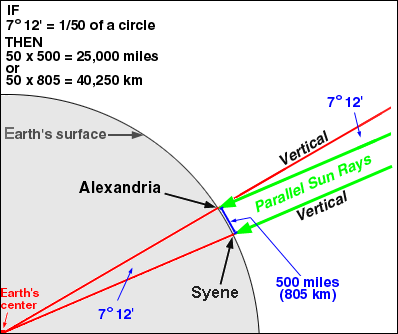I thought it might be fun to see if we can reproduce this result using some helpful sites on the web.

SUNCALC.ORG

This website lets you choose any location on the planet, choose the date you want to see, and then watch how an object’s shadow changes throughout the day.  So you can have your students look for a place and time when the object’s shadow disappears!  (You can provide more or less guidance, depending on how much time you want to spend on this activity.)

Here is a screenshot showing the shadow length of a 1 m tall object in Sombrerete, Mexico on June 21st: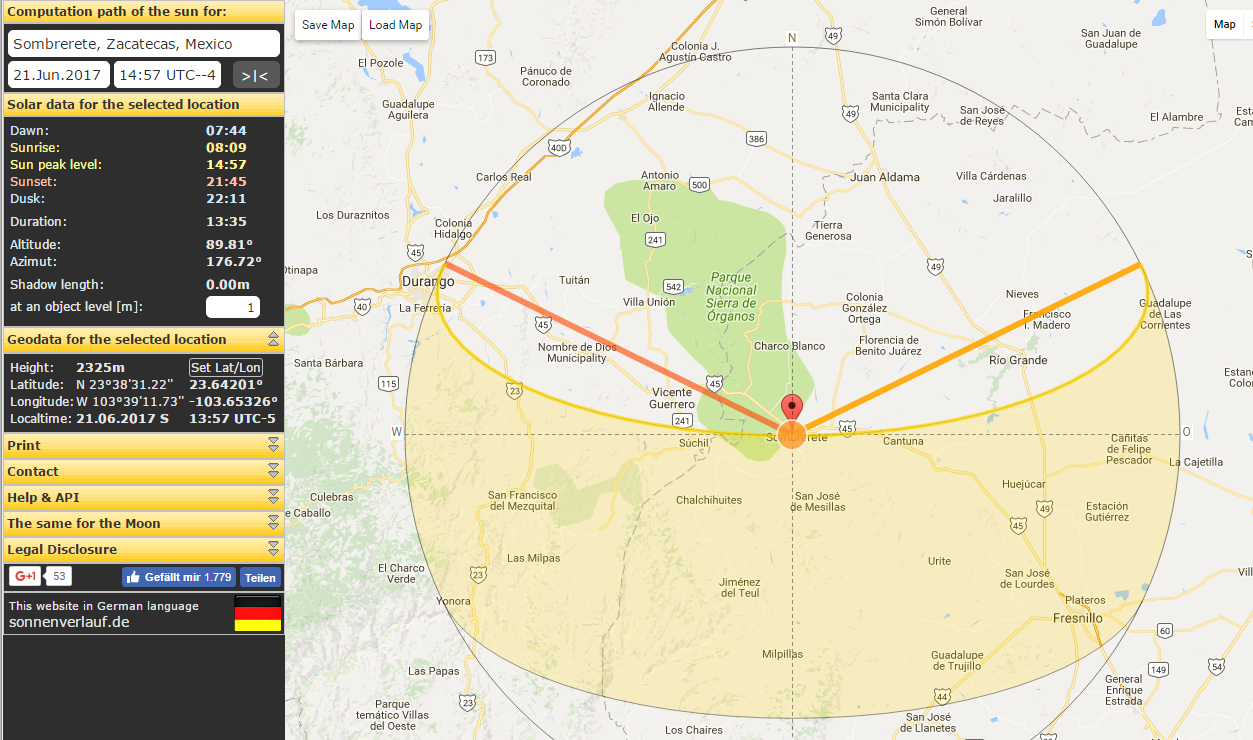You can see that the shadow is gone!  Depending on how much you have revealed to your students, it might be helpful to note the latitude (in the window on the lower left).

The next step is to choose another location north or south of our first location so that we can check out the shadow there.  I went north to the little town of Olney Springs in Colorado.  I chose this pretty much at random but nearly due north from Sombrerete.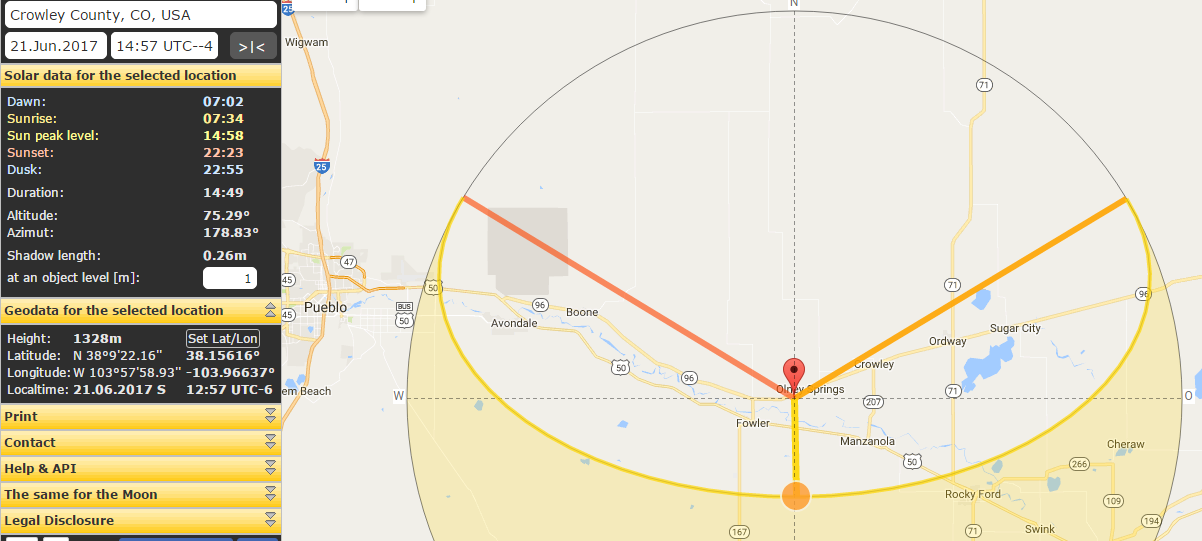You can see that on the same time and date as before, the shadow of the 1-m tall object is now .26 meters.  You can use the inverse tangent to get the angle of the sun.  Or, if you feel lazy, you can read the angle right from the website — but that is the complement of the angle we need.  Either way, you get 14.7 degrees.

Now we just need to know how far it is from Sombrete to Olney Springs.  Google Maps to the rescue:That’s 1,614 km, nearly due north.  And it corresponds to a central angle of 14.7 degrees.  We are almost home free: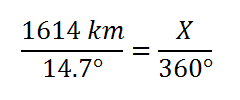This solves to give as the circumference of the Earth: 39,526 km.

From there, we get a radius of 6,290 km — a result that is less than 2% away from the “official” value.

Of course, it’s good to remind students that Eratosthenes worked this out more than two millennia ago!

# Alternative Solutions: Practice Test #6

DITW = Do it their way

Trial and Error = pick an answer, play with it, see if it fits what they said in the problem…

Back Door = make up numbers for the variables in the problem, work out an answer that is based on the numbers you made up, then put those made up numbers back into the answer choices, ruling out any that don’t produce a matching answer.

More detailed explanations available in the book…

PRACTICE TEST #6, SECTION 3 – NO CALCULATOR

1. Linear modeling again!  When y=mx + b (or b + mx) the b is the starting value.  In this case, that’s the service fee.
2. DITW
3. DITW
4. You don’t have to FOIL this out all the way.  Just notice that the x^2 term has to be p^2…
5. Yes, you should be able to match a graph and its equation.  But if you can’t…pick any x value,,,say x=2.  Find the y-value that goes with that x value.  (You get y=-1) .  Then, check which graph contains the point you have just found, (2,-1).
6. DITW
7. If you need to, use the back door: make up numbers, then put your numbers into the answer choices to see which one works.
8. No real trick to this one once you understand what they are asking.   In other words, when you add entry in the second column to the one in third column,  do you get the value in the first column?
10. DITW
12. They want you to do polynomial division.  But seriously, this one cries out for the back door.  Try letting x=1.  Work out the answer.  Then put x=1 into each answer choice.  It’s much easier my way.
13. This is actually pretty subtle.  You could try each value and see if it factors (or see if the discriminant is negative). That’s kind of a pain.  There is another way to get the answer but I admit it is sneaky.  Consider the quadratic function y=2x^2-4x-t.  It opens upward.  So if it has no real solutions, if you shift it upward it will still have no real solutions.  But the problem can only have one answer!  So it has to be A.  (If this doesn’t make sense to you, post a question and I will go into it in more detail.  Also, I predict that very few test-takers will get this one right.)
14. DITW
15. Try numbers!  I used a=1 and b=4 so I got 9.  But oh, no!  A and D both come out to 9.  No worries – just do it again with new numbers. I tried a=1, b=6 and got 16.  This time, only D worked.
16. Other than playing around with numbers, how else are you going to do this?
17. DITW
18. This is a Pythagorean Theorem question.  But first, when you see this shape you should automatically be on the alert for similar triangles and ratios…
19. DITW – even I admit that algebra is the fastest way.  But if you are comfortable with the idea of weighted averages and inverse proportions, there is another way to think about this one.

The goal is a 15% solution. Since that is closer to the 10% stuff than it is to the 25% stuff, I expect to need less of the 25% solution than the 3 liters I already have.  In fact, if you look at how far each solution is from the goa, the 10% stuff is 5% away, the 25% stuff is 10% away.  Like weights balancing on a see-saw, the farther away, the greater the effect.  In fact, it is an inverse proportion.  So I know I’ll need half as much of the 25% solution.

OK, I admit that if I were taking this test, I would never have come up with that method on time.  So in this case, do the algebra if you can.  If not, take a guess and move on.

1. DITW

PRACTICE TEST #6, SECTION 4 – WITH CALCULATOR

1. Combine like terms if you want.  Or try a number!
2. DITW
3. You are looking for shallow, then level, then steep.  Slope is rate of change.  Also, the snow was always getting deeper so C and D are silly.
4. DITW
5. They are just checking if you know that you can divide both sides of an inequality, in this case by 3.
6. DITW
7. It seems from the released tests that the SAT would really like you to know that to make accurate predictions, a survey must involve RA NDOM sampling.
8. If this is your sixth practice test, then you have seen this before.  But read carefully so you know if they are asking about everybody or just a subset.  This time, they only want to know about vanilla lovers.
9. DITW
10. By algebra, if x is the second voyage, then x + x + 43 = 1003.  But why not just try the answer choices?
11. DITW
12. You don’t have to calculate the average growth rates to see which is greatest.  Average growth rates are also slopes of the segments that connect the two points.  So draw them and then pick the steepest one.
13. And here we have yet another linear model: y= mx + b but with some of the letters changed.  The a value is still the rate of change, or in this case, the amount of daily growth.
14. Pick a value from the graph or chart . For instance, on day 28 the height was 98.  Try t = 28 in each answer choice.  You will see that only one answer is even close.
15. Really?  The very next question?  I tried x = 4…
16. These are similar triangles.  It might help you to see that better if you redraw the one on the left, turning it and flipping it so that it is oriented the same way as its neighbor…
17. Make up a set of numbers so that 2h + d = 25.  Then go to the answers…by now you should know that I call that “the back door play”.  In basketball, the back door play gets you an easy layup.
18. DITW
19. Trial and error!  For example, if d=7.2 inches then 2h = 25-7.2=17.8 inches so h=8.9 inches.  No good.  If it had fallen in the right range, we would then check if the number of steps came out odd.  Keep going until you find the one that works…
20. DITW
21. DITW
22. Many people find it helpful to list out the data:

10,10,10,10, 11,11,11,11,12,13,15,15,15,17,20,21,21,27,31,34,55

Once you do that, finding the median is just a matter of finding the middle number.  Also, once you have done a few this way, you won’t need to any more.  You can just think: there are 21 states on the list so 10 of them will be greater than or equal to the median, 10 will be less than or equal to the median and the 11th one will be the median.  Start at the top of the chart and keep a running tally of how many states you have accounted for.  By the fourth row, you will have counted 10 states.  The median will be in the next row.

1. DITW
2. You can DITW or you can use trial and error with each answer choice.
3. Linear functions are “evenly spaced”.  So to start,  figure out what goes between the first row and the second row.  Then you will know how much you have to go up by to get to f(3).
4. DITW
5. DITW
6. This is yet another example where you are better off finding your own answers first and then checking which equation matches them.  Just draw a number line and you can easily see that the two points they are talking about are -1 and -7.  So check each answer to see which one works with both of those values.
7. Let’s make up numbers again.  For example, if t= 25, then s = 80 and the average speed is 80/25=3.2 inches per second.  Now, it’s off to the answer choices!
8. Hmm..if we drew a curve through these points it would be a downward parabola.  So you know it is B or D.  When x=4, it looks like y= 800 or so.  Plug in x=4 into B and D.  It won’t be a close call.
9. Just try numbers…easy numbers.
10. DITW
11. DITW…remember the formulas are given in the front of the section
12. If you don’t want to do the algebra, you can graph both functions on a graphing calculator and then trace along until you come to the intersection point.
13. DITW
14. If the average is .1 greater and there are 6 items then the total is .6 greater…
15. Make a little chart, fill in the 480 dollars at the bottom,  then work your way back up, cutting the value in half each time.
16. Here’s a hint: the 6 students represent 15% of the committee.  You can set up ratios to find the rest of the committee members.  For example, to find the administrators:  6/15 = x/25